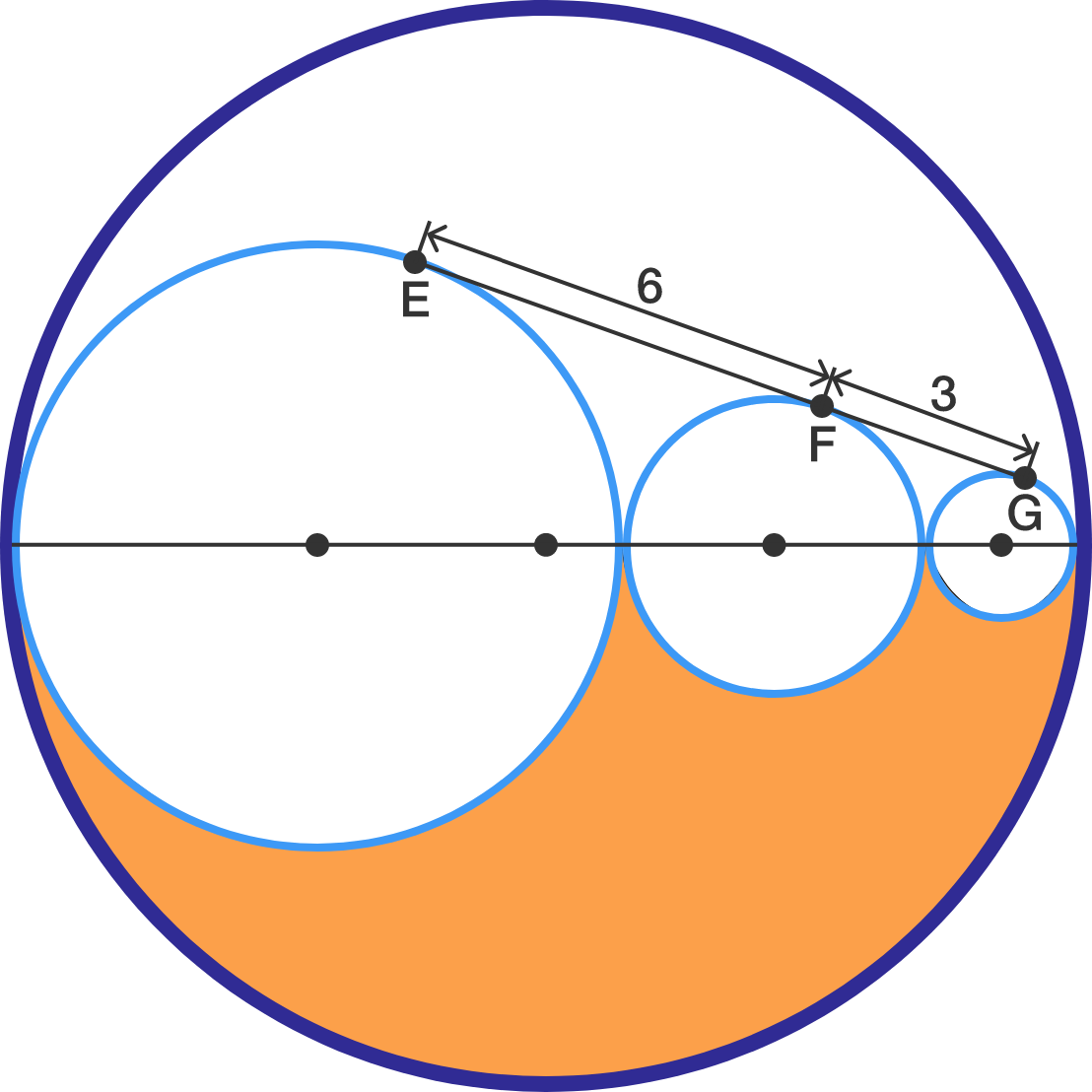What A Weird Shape!

Geometry Level 3There is a common tangent which intersects the three tangent circles shown above at $E,$ $F,$ and $G.$ If $EF = 6$ and $FG = 3$, find the area of the orange region. Use the approximation $\pi \approx \frac{22}{7}.$

×